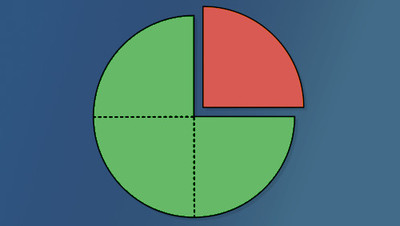# Basic Math (Arithmetic)

Educator Edition

 Basic Math (Arithmetic). Covers fractions, decimals, percents, integers, and measurements to write, manipulate, interpret, and solve application and formula problems. Explores some measures of central tendency. A scientific calculator is required. The TI-30X II is recommended.

## Customize your Student Experience

Edfinity is a full-featured homework system. Use this problem series as-is, or mix-and-match with other series or your own problems as desired. Edfinity gives you complete control over the every aspect of students' online assessment experience.

## Intended Outcomes

Upon completion of the course students should be able to:

• Choose and perform accurate arithmetic computations in a variety of situations with and without a calculator.
• Creatively and confidently apply mathematical problem solving strategies.

## Powered by WeBWorK

This problem series includes WeBWorK-enhanced problems. WeBWorK problems are highly sophisticated, supporting the representation and manipulation of mathematical objects such as vectors, points, matrices, intervals, and complex numbers, with customizable answer checkers. Students are encouraged to make multiple attempts until they succeed, and given immediate feedback on correctness.

Edfinity provides full access to the WeBWorK Open Public Library (OPL), a collection of over 20,000 rich, interactive problems across a wide range of math and science subjects, including college algebra, discrete mathematics, probability and statistics, single and multivariable calculus, differential equations, linear algebra and complex analysis.

## Problem Sets

1. Edfinity Demo
2. Exponents And Rounding Whole Numbers
3. Multiplication
4. Division
5. Geometry Basics
6. Divisibility Test
7. Prime Numbers
8. Gcf And Lcm
9. Order Of Operations 1
10. Integers And Absolute Value
11. Add Subtract Integers
12. Multiply Divide Integers
13. Order Of Operations 2
14. Fraction Definition
15. Add Subtract Fractions
16. Multiply Fractions
17. Divide Fractions
18. Mixed Number Definition
19. Add Subtract Mixed Numbers
20. Multiply Divide Mixed Numbers
21. Order Of Operations 3
22. Decimal Definition
23. Decimal Addition Subtraction
24. Decimal Multiplication Division
25. Decimal And Fraction
26. Square Root
27. Mean Median Mode
28. Rate And Ratio
29. Proportion
30. Unit Conversion
31. Percent Definition
32. Percent Formula
33. Percent Word Problem
34. Percent Of Increase Decrease

##License

This is a multi-student, educator license. You will be able to manage an online course with your students, and monitor their progress.

Price: FREE
Trusted by## Recommendations

Cookies help us deliver our services. By using our services, you agree to our use of cookies.